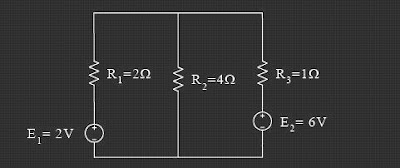## Tuesday, July 21, 2009

### Analisis Loop/Mesh Pada Rangkaian Elektronika

Stages to implement the Loop Analysis / Mesh is this:
1. Determine the direction of flow in each loop in the series. Usually selected with the clockwise direction.
2. Determine the polarity of each element in the series.
a. In the source: cash flow from the polarity (-) to the polarity (+)
b. In the burden: cash flow from the polarity (+) to the polarity (-)
3. Apply Kirchoff Voltage Law in each loop is in series, so that equality of the number of the loop.
4. Complete the simultaneous equation.

Example
Decide which is the current flowing in each loop of the following series!Skema Rangkaian Elektronika